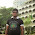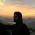## Electrical DC Circuits Objective Questions with Answers:

 Suppose Double the voltage in a simple dc circuit and cut the resistance in half , then the current will
(a) Become four times as great
(b) Become twice as great
(c) Stay the same as it was before
(d) Become half as great

 If four resistors are connected in series, each with a value of 4Kohm, the total resistance is
(a) 1kΩ
(b) 4 kΩ
(c) 8 kΩ
(d) 16 kΩ

 Three resistors each with a value of 0.069M ohm are in parallel. The total resistance is
(a) 23 Ω
(b) 23 KΩ
(c) 204 Ω
(d) 0.2 mΩ

 Proper biasing in an amplifier circuit
(a) Causes it to oscillate
(b) Prevents an impedance match
(c) Can be obtained using a voltage divider network
(d) Maximizes current flow

 A transistor can be protected from needless overheating by
(a) A current-limiting resistor
(b) Bleeder resistors
(c) Maximizing the drive
(d) Shorting out the power supply when the circuit is off

 A bleeder resistor
(a) Is connected across the capacitor in a power supply
(b) Keeps a transistor from drawing too much current
(c) Prevents an amplifier from being over driven
(d) Optimizes the efficiency of an amplifier

 An advantage of rheostat over a potentiometer is
(a) Rheostat can handle higher frequencies
(b) Rheostat is more precise
(c) Rheostat can handle more current
(d) Rheostat works better with dc

 The average power delivered to an impedance (4 - j3)Ω  by a current 5cos(100
πt + 100π)A is [GATE 2012]
(a)  44.2W
(b)  50W
(c)  62.5W
(d) 125W

 A periodic voltage waveform observed on an oscilloscope across a load is shown. A permanent magnet moving coil (PMMC) meter connected across the same load reads [GATE 2012]
(a) 4 V
(b) 5 V
(c) 8 V
(d) 10 V

The bridge method commonly used for finding mutual inductance is
[GATE 2012]
(a) Heaviside Campbell bridge
(b) Schering bridge
(c) De Sauty bridge
(d) Wien bridge

Solution Hint: Heaviside-campbell equal ratio bridge is used for measuring self-inductance in terms of mutual inductance. Schering bridge = used for measuring capacitance and dielectric loss of a capacitor. De Sauty bridge = used for comparing the capacitances of two capacitors. Wien bridge = used for audio-frequency measurement of capacitors over a wide range.

 An ideal voltage source will charge an ideal capacitor[Gate 1997]
(a) In infinite time
(b) Exponentially
(c) Instantaneously
(d) None of the above

 A practical current source is usually represented by[Gate 1997]

(a) A resistance in series with an ideal current source
(b) A resistance in parallel with an ideal current source
(c) A resistance in parallel with an ideal voltage source
(d) None of the above

 Energy stored in a capacitor over a cycle, when excited by an ac source is[Gate 1997]

(a) The same as that due to a dc source of equivalent magnitude
(b) Half of that due to a dc source of equivalent magnitude
(c) Zero
(d) None of the above

 A major advantage of the active filters is that they can be realized without using[Gate 1997]

(a) Opamps
(b) Inductors
(c) Resistors
(d) Capacitors

 The computer program which converts statements written in high level language to object code is  known as [Gate 1997]

(a) Assembler
(b) Operating system
(c) Object oriented software
(d) None of the above

1.2.